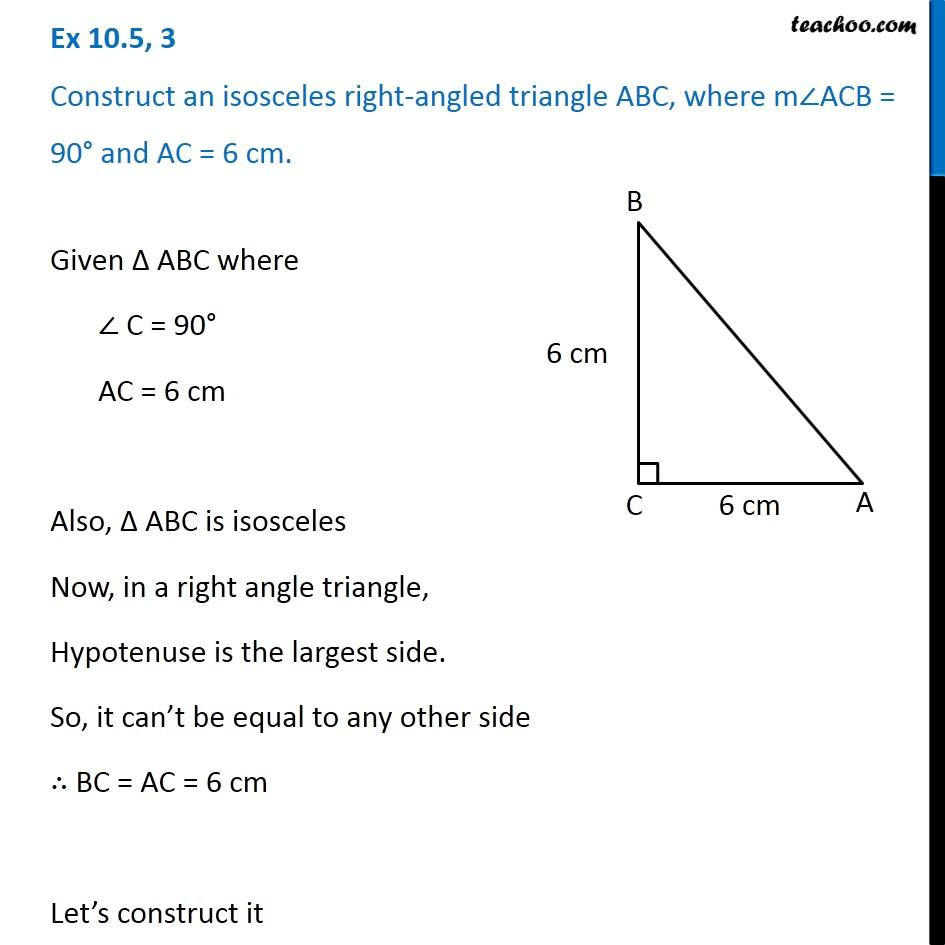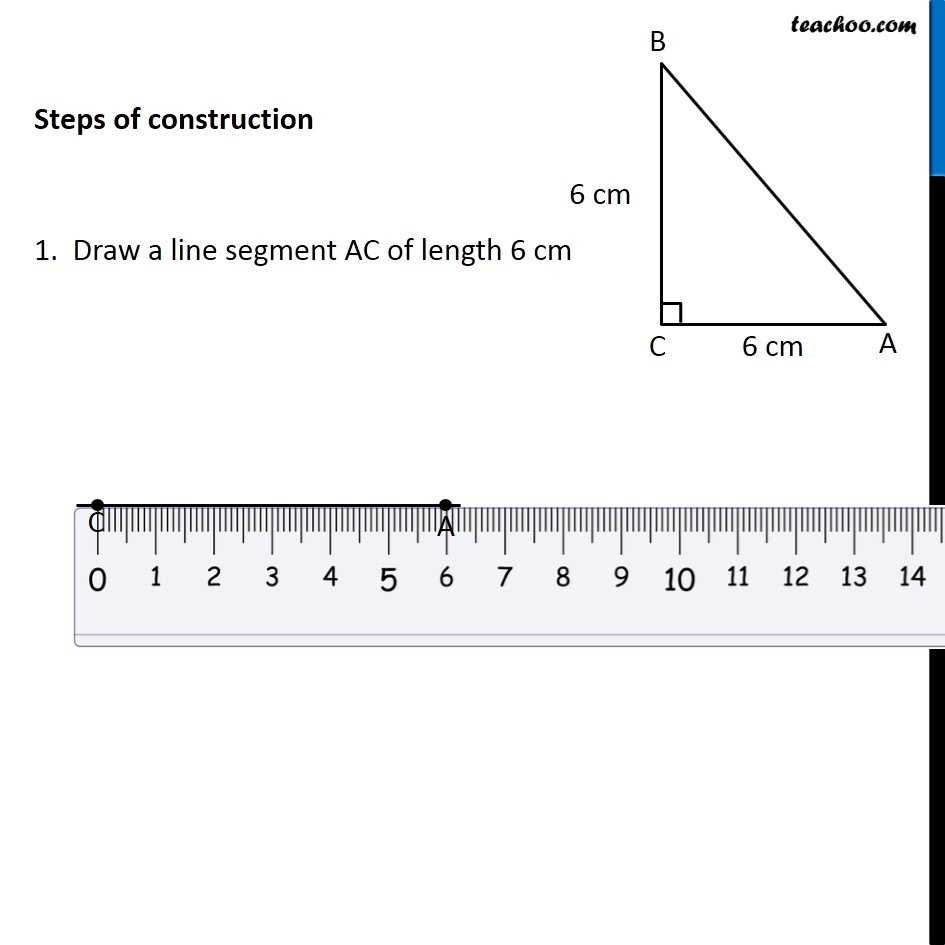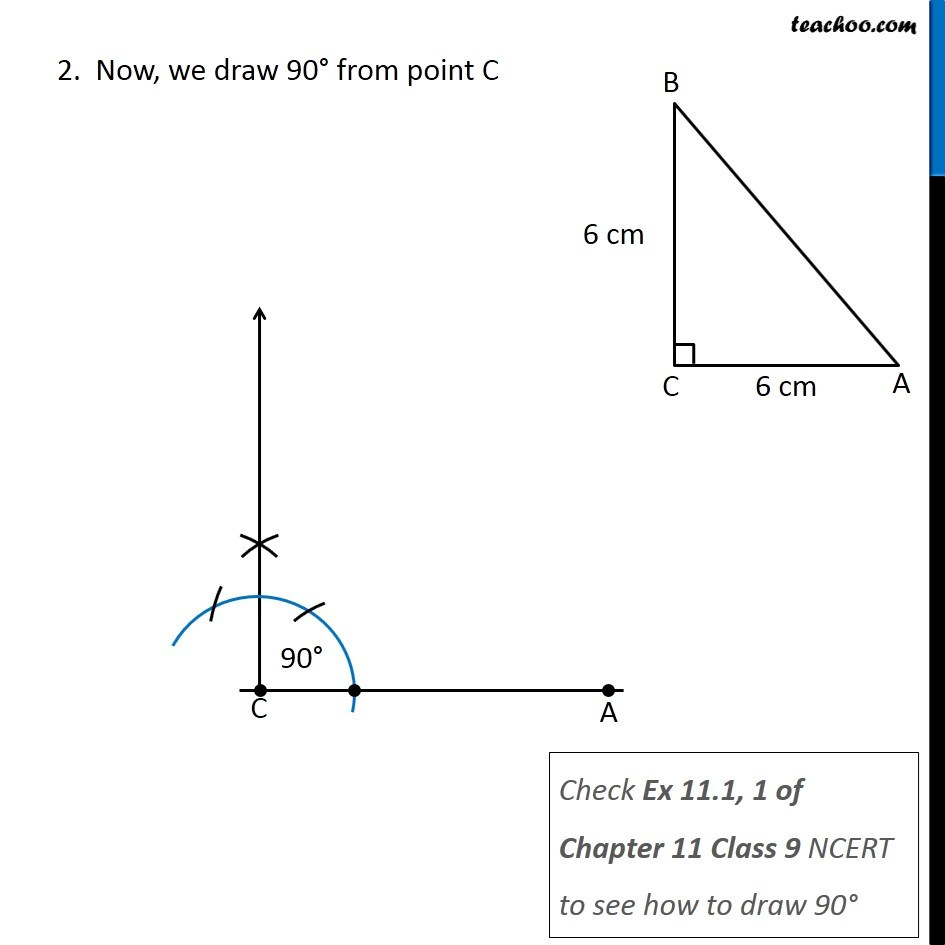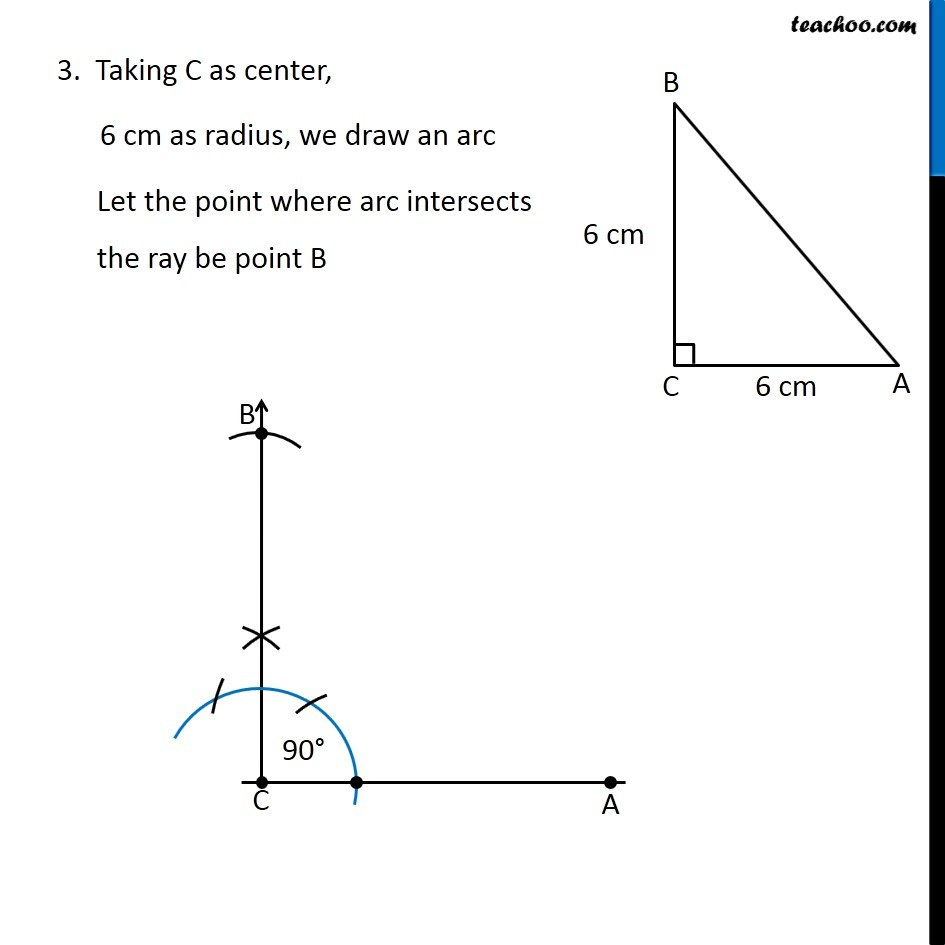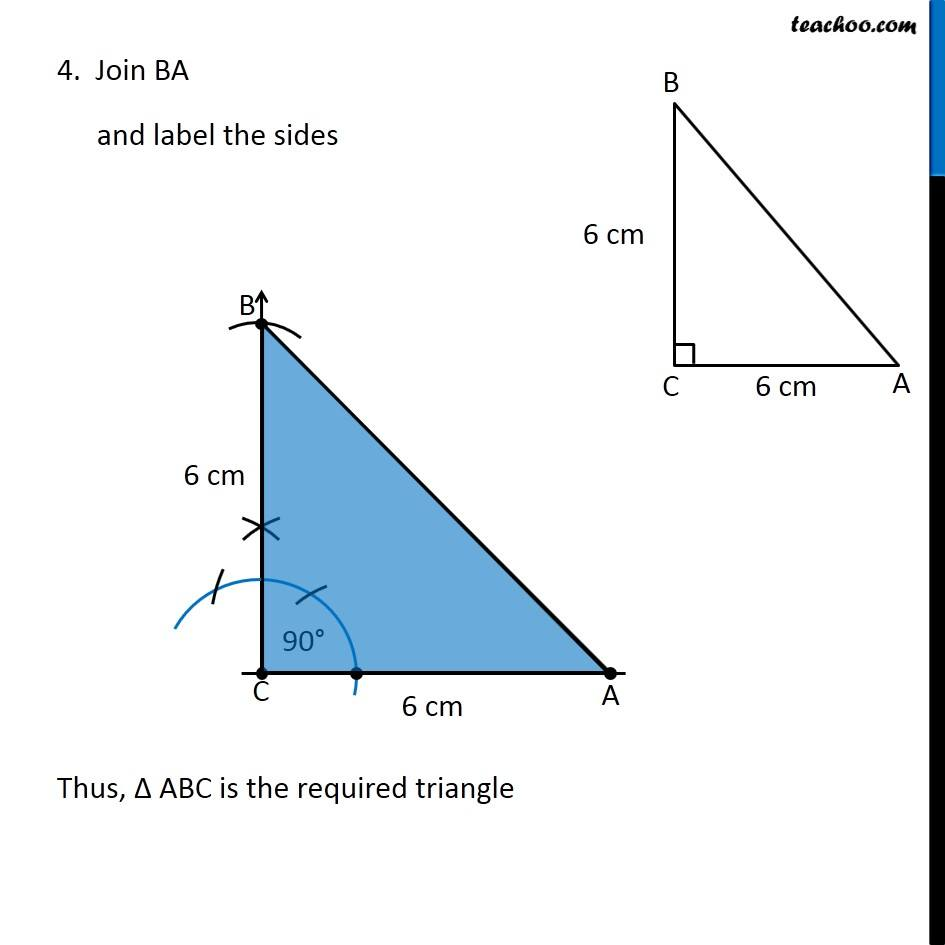1. Chapter 10 Class 7 Practical Geometry
2. Concept wise
3. Constructing a right triangle when hypotenuse and side is given (RHS)

Transcript

Ex 10.5, 3 Construct an isosceles right-angled triangle ABC, where m∠ACB = 90° and AC = 6 cm. Given Δ ABC where ∠ C = 90° AC = 6 cm Also, Δ ABC is isosceles Now, in a right angle triangle, Hypotenuse is the largest side. So, it can’t be equal to any other side ∴ BC = AC = 6 cm Let’s construct it Steps of construction 1. Draw a line segment AC of length 6 cm 2. Now, we draw 90° from point C Check Ex 11.1, 1 of Chapter 11 Class 9 NCERT to see how to draw 90° 3. Taking C as center, 6 cm as radius, we draw an arc Let the point where arc intersects the ray be point B 4. Join BA and label the sides Thus, Δ ABC is the required triangle